# Study of Number Systems

In this article we will have a detailed study of number systems, their presentation and conversion from one number system to another.

A number system is a code that uses symbols to count the number of items.

#### Decimal Number System:

The decimal number system is the most commonly and widely used number system. It uses the symbols 0,1,2,3,4,5,6,7,8 and 9. Thus, the decimal system has ten digits for counting the items.

Since there are ten digits in this group, we say the number system has a base or radix of 10. In the decimal number system, numbers greater than 9 are represented by repeatedly using these digits in a definite order, as illustrated below:

Consider the decimal number 754. We may write this as (754)10, where the subscript 10 shows that 754 is a decimal number. This is a short form of writing, which may be expanded as:

Where 7, 5 and 4 are the coefficients of the appropriate powers of ten (i.e. 10­­2, 101 and 100 respectively).

Larger numbers can be expressed similarly by writing them as coefficients of the powers of ten.

Let us now consider a situation wherein we want to represent the decimal numbers using electrical signals. To do so, we require a switch, which can assume ten different voltage levels. However, at present, there is no electric switch that can assume ten different voltage levels. Hence we are unable to use the decimal number system as the number system on which logic and switching circuits and systems are based. This is the major disadvantage of the decimal number system.

#### Binary Number System:

Consider an ordinary electric switch. As we know that this switch has two states of operations: ON and OFF. If we assign 0 to the OFF – state and 1 to the ON- state, then we can use the switch to represent the numbers system, which has digit 0 and 1 as its basic members. This number system, which has 0 and 1 as its basic members, is known as the binary number system.

We may replace mechanical switches with electronic switches such as diodes and transistors. Electronic switches have several advantages over mechanical switches such as:

1. Electronics switches are extremely fast.
2. They occupy very small space.
3. Cost of an electronic switch is much lower than that of a mechanical switch.
4. Electronic switches are available on integrated-circuit form.
5. Complex logic functions can be easily realized by using electronic switches.

Hence, the binary number system is the most suitable number system to represent logic functions.

#### The Binary Number System

The binary number system uses a radix of 2. The members of this number system are 0 and 1 and these are used to represent any number in this number system. For example (10110)2 represents a binary number, where the digits 1,0,1,1 and 0 represents coefficients of appropriate powers of 2. The number (101101)2 may be expanded as below:= 22

The decimal equivalent of this number is obtained by converting the powers of 2 to their decimal values. Thus;Using this procedure we can convert any binary number into its equivalent decimal number.

#### Conversion from Decimal into Binary:

There are two methods for converting decimal numbers into their corresponding binary numbers.

• Method-1: By Actual Division

Example : Convert the decimal number 29410 into its binary number equivalent.

Solution: Divide the given number repeatedly by 2.

In each division, if the number is exactly divisible by 2, we put a 0 as remainder against the number on its right-hand side.

If the number is not exactly divisible by 2, we put a 1 as the remainder.

We proceed like this up to the last digit.

Then we read upwards as shown by the direction of arrow, to get the required binary number.

 Number 294 divide by 2 result 147 remainder 0  (LSB) divide by 2 result 73 remainder 1 divide by 2 result 36 remainder 1 divide by 2 result 18 remainder 0 divide by 2 result 9 remainder 0 divide by 2 result 4 remainder 1 divide by 2 result 2 remainder 0 divide by 2 result 1 remainder 0 divide by 2 result 0 remainder 1  (MSB)

The binary result is obtained by placing all the remainders in order with the least significant bit (LSB) being at the top and the most significant bit (MSB) being at the bottom.

Hence,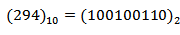• Method-2: By tabulation

Example: Convert (88)10 into binary.

To solve the problem by tabulation method, we write the numbers in a horizontal manner, as shown below. We write 88 in the right-most position of the first row.

We then process in the direction of arrow shown, and divide each number by 2.

If the number is fully divisible by 2, we write 0 (otherwise a 1) below each number.

We proceed until the last digit is arrived at.

1                 2                  5                  11             22               44                88        Number

2                 2                  2                   2               2                  2                  2          Radix

0                1                  2                   5                11              22               44          Quotient

1                0                  1                   1                 0                0                 0           Remainder

The desired binary number can now be read from left to right as 1011000.

Hence, (88)10 = (1011000)2

#### Decimal and Binary Fractions

In the decimal system fractions are represented as 0.xxx, where x’s represent digits from 0 to 9. The decimal point separates the integral part of the number from its fractional part.

Consider the number (58.1012)10. The expansion of this number is as below:

Integral part: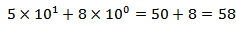Fraction :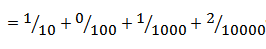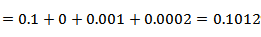The numbers after the point symbol are expanded by using negative powers of 10. By using a similar method, we can express binary fractions.

Let’s take an example of binary number (101.1101)2. By expanding it we get,

Integral part: 101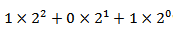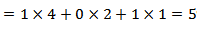Fraction :0.1101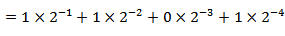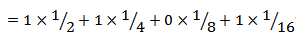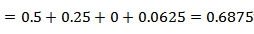The decimal equivalent of this binary then becomes 5.6875

The point symbol in the binary system is called as the binary point or radix point.

#### Conversion of Decimal Fraction into Binary

Just as we divide a decimal whole number by the factor 2 to get its binary-equivalent, to get the corresponding binary fraction we multiply the decimal fraction by 2. If the product of each number is greater than 1, we put a 1 below that number; otherwise we put a 0. This is shown in the following example.

Example: Convert (0.625)10 decimal number to binary number (?)2 using successive multiplication method

1st Multiplication Iteration
Multiply  0.625  by  2                 0.625 × 2 = 1.25(Product)         Fractional part=0.25            Carry=1    (MSB)
2nd Multiplication Iteration

Multiply  0.25  by  2                      0.25 × 2 = 0.50(Product)         Fractional part = 0.50            Carry = 0

3rd Multiplication Iteration

Multiply 0.50  by  2                      0.50 x 2 = 1.00(Product)         Fractional part = 1.00            Carry = 1  (LSB)

The fractional part in the 3rd iteration becomes zero and hence we stop the multiplication iteration.
Carry from the 1st multiplication iteration becomes MSB and  carry from 3rd iteration becomes LSB.

Hence, the fractional binary number of the given fractional decimal number (0.625)10 is (0.101)2.

#### The Octal Number System

The octal number system contains the whole numbers from 0 to 7. In this system, the base or radix is 8.

For example, a number 127 is a valid octal number. The decimal equivalent of (127)8 is given by:

 Octal Digit Value 127 In polynomial form = ( 1×82 ) + ( 2×81 ) + ( 7×80 ) Add the results =  ( 64 ) + ( 16 ) + ( 7) Decimal number form equals:   8710

Then, converting octal to decimal shows that  1278 in its Octal form is equivalent to 8710 in its Decimal form.

#### Conversion of Decimal into Octal:

In this post, we will see the step-by-step procedure to convert given decimal number to its equivalent in the octal number system.

As discussed earlier, we will implement the same division method used earlier, to convert decimal to octal and all the steps will be very much similar to the steps shown in decimal to binary conversion except for the change in the radix from 2 to 8 since here the target number system is octal number system.

1. Integer part of the given decimal number is successively divided by the radix(base) of the target number system 8(octal) until we reach a stage where the quotient becomes zero(0).
2. The reminder obtained at the end of each division step, becomes a part of the octal number.
3. The reminders that obtained at the end of each step are assigned weights in the octal number system starting from the reminder obtained at the last division iteration to the first division iteration in decreasing order.
4. The reminder in the last division iteration has the highest weight (MSB) and the reminder obtained in the first division iteration has the lowest weight (LSB) in the octal number system.
5. The procedure is illustrated in the example below:

Example: Convert the decimal number (87)10 into octal.

Solution:

1st Division Iteration

Divide 87 by 8                       87 ÷ 8 = 10(Quotient)                    Reminder:7

2nd Division Iteration

Divide 10 by 8                         10 ÷ 8 = 1(Quotient)                     Reminder=2
3rd Division Iteration

Divide 1 by 8                            1 ÷ 8 = 0(Quotient)                      Reminder=1

Remainder from the last division iteration becomes MSB and reminder from 1st iteration becomes LSB.

So the octal equivalent of 8710 is 1278

#### Conversion of Octal into Binary:

To convert an octal number into its corresponding binary, first we have to convert it into its decimal equivalent and then the decimal number is converted into binary.

Let’s take an octal number (127)8. To convert this into binary, we first convert (127)8 to decimal, which is equal to (87)10 as explained before.

Now (87)10  is converted into binary as described above.

So we get (87)10 = (1010111)2

This procedure is lengthy and time consuming. So there is an easy and faster method to convert octal to binary.

For this, first we write the octal number with some spacing between the digits as :

1            2            7

Now, below each number, we write its binary equivalent in 3bit form. We then get

1             2            7

001         010      111

Finally combining all the bite, we have

(127)8 = (001010111)2 = (1010111)2

#### Conversion of Binary into Octal

For converting a binary number into octal, we divide the binary number into groups of 3 bits each, starting from the right-most bit and then write the corresponding decimal number below these groups. Then the decimal number is converted into its octal equivalent.

Example: Find the octal equivalent of (1011011101)2

Solution: Proceeding as described above, we first divide the number into groups of 3 bits each.

So we have the following groups:

1               011                011                  101

We  now add zeros before the left-most 1 to complete the groupings, and write the corresponding decimal numbers below each group. Thus, we get

001         011          011            101

1             3               3                5

Hence, (1011011101)2 = (1335)8

#### Octal Fractions

Octal fractions can be obtained by using procedures similar to those developed for binary fractions.

Example: Convert (21.21)8 to decimal.

Solution:

21.21

=  2 × 81 + 1 × 80 + 2 × 8-1 + 1 × 8-2
= 2 × 8 + 1 × 1 + 2 ×( 1 / 8 ) + 1 × ( 1 / 64 )
= 16 +  1  +  ( 0. 2 5 ) +  ( 0 . 0 1 5 6 2 5 )
= 17 +  0. 265625

= 17 . 265625

Therefore  ( 2 1 . 2 1 )8 =  ( 1 7 . 2 6 5 6 2 5 )10

#### Conversion of Decimal Fraction into Octal

Example: Convert (49.21875)10 to octal.

Solution:

The conversion is done as shown in the following table. We have divided the number into its integral and fractional parts.

Integral Part :49                        Fractional Part:0.21875

Integral part:

1st Division Iteration

Divide 49 by 8                       49 ÷ 8 = 6(Quotient)                    Reminder:1 (LSB)

2nd Division Iteration

Divide 6 by 8                         6 ÷ 8 = 0(Quotient)                     Reminder=6  (MSB)

So the octal equivalent of 4910 is 618

Fractional part: 0.21875

.021875
×         8
1.75000  —–> 1 (MSB)

.75
× 8
6.00 — —–> 6 (LSB)

So the octal equivalent of 0.2187510 is 168

Hence, we get     (49.21875)10 = (61.16)8

The hexadecimal number system uses a base or radix of 16. Digits range from 0 to 15 in this system; these are shown in table 1. Here in addition to digits from 0 to 9, alphabets A,B,C,D,E and  F are used to represent hexadecimal numbers 10,11,12,13,14 and 15 respectively.

Table – 1 Representation of Hexadecimal Numbers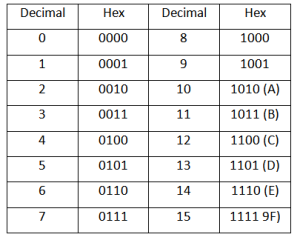#### Conversion of Decimal into Hexadecimal

Solution:

1st Division Iteration

Divide 249 by 16                          249 ÷ 16 = 15(Quotient)                    Reminder: 9 (LSB)

2nd Division Iteration

Divide 15 by 16                                15 ÷ 16 = 0(Quotient)                  Reminder= 15(F)  (MSB)

So the Hexadecimal equivalent of 24910 is (F9)16

Example: Convert (567.1875)10 into hexadecimal(or hex).

Solution:  We have

Integral Part: 567                                                  Fractional: 0.1875

Integral Part:

1st Division Iteration

Divide 567  by 16                      567 ÷ 16 = 35(Quotient)                    Reminder: 7 (LSB)

2nd Division Iteration

Divide 35 by 16                                35 ÷ 16 = 2(Quotient)                  Reminder= 3

3rd Division Iteration

Divide 2  by 16                                 2 ÷ 16 = 0(Quotient)                   Reminder: 2 (MSB)

Hence  Hexadecimal equivalent of 56710 is (237)16

Fractional Part: 0.1875

.1875
×   16
3.0000  —–> 3

So (875)10 = (0.3)16

Hence, we get   (567.1875)10 = (237.3)16

#### Conversion of Hex into Direct Binary and Back

As we take group of 3 bits for octal, so for hex, groups of 4 bits are taken.

Example: Find the binary equivalent of (F9)16.

Solution:

We have

F                   9

1111              1001

That is    (F9)16 = (11111001)2

Example: Convert (1111001)2 to hex.

Solution:

For hex, groups of 4 bits are taken as

0111                  1001

7                          9

Thus,  (1111001)2 = (79)16